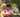### Genomic Range Query

January 26, 2020

Genomic Range Query

A DNA sequence can be represented as a string consisting of the letters A, C, G and T, which correspond to the types of successive nucleotides in the sequence. Each nucleotide has an impact factor, which is an integer. Nucleotides of types A, C, G and T have impact factors of 1, 2, 3 and 4, respectively. You are going to answer several queries of the form: What is the minimal impact factor of nucleotides contained in a particular part of the given DNA sequence?

The DNA sequence is given as a non-empty string S = SS...S[N-1] consisting of N characters. There are M queries, which are given in non-empty arrays P and Q, each consisting of M integers. The K-th query (0 ≤ K < M) requires you to find the minimal impact factor of nucleotides contained in the DNA sequence between positions P[K] and Q[K] (inclusive).

For example, consider string S = CAGCCTA and arrays P, Q such that:
P = 2 Q = 4
P = 5 Q = 5
P = 0 Q = 6

The answers to these M = 3 queries are as follows:
• The part of the DNA between positions 2 and 4 contains nucleotides G and C (twice), whose impact factors are 3 and 2 respectively, so the answer is 2.
• The part between positions 5 and 5 contains a single nucleotide T, whose impact factor is 4, so the answer is 4.
• The part between positions 0 and 6 (the whole string) contains all nucleotides, in particular nucleotide A whose impact factor is 1, so the answer is 1.

Write a function:
function solution(S, P, Q);
that, given a non-empty string S consisting of N characters and two non-empty arrays P and Q consisting of M integers, returns an array consisting of M integers specifying the consecutive answers to all queries. Result array should be returned as an array of integers.

For example, given the string S = CAGCCTA and arrays P, Q such that:
P = 2 Q = 4
P = 5 Q = 5
P = 0 Q = 6
the function should return the values [2, 4, 1], as explained above.

Write an efficient algorithm for the following assumptions:
• N is an integer within the range [1..100,000];
• M is an integer within the range [1..50,000];
• each element of arrays P, Q is an integer within the range [0..N − 1];
• P[K] ≤ Q[K], where 0 ≤ K < M;
• string S consists only of upper-case English letters A, C, G, T.

Testcase : ('CAGCCTA', [2, 5, 0], [4, 5, 6])

``````function minImpact(str){
if(str.includes('A')) return 1
if(str.includes('C')) return 2
if(str.includes('G')) return 3
return 4
}

function solution(S, P, Q) {
// write your code in JavaScript (Node.js 8.9.4)
let res = []
let str = []
for(let i=0; i<P.length; i++){
str = S.slice(P[i], Q[i]+1)
res[i] = minImpact(str)
}

return res
}``````Posted by Tai Lu ( 呂台生 ) who's building useful things.You should follow him on Twitter